Home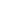Courses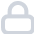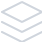Assessments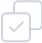Workshops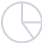Job PostingLMSShare

Glad you have shown interest in learning Basic introduction to FEA | Skill-Lync. Share this with a friend.Close# Turbulence and CFD

Master the core concepts of CAE engineering with the free FEA online course. In this free CFD course, our instructor clearly explained the different methods for solving engineering problems. Towards the end of this course, you will understand the different types of FEA, key concepts and career oppor... See moreIntroduction to Basic introduction to FEA

This video gives an introduction to the course, Basic introduction to finite element analysis, the need for FEA, and the numerical method of solving engineering problems is explained in this video.

06:41

Introduction to Basic introduction to FEA

This video gives an introduction to the course, Basic introduction to finite element analysis, the need for FEA, and the numerical method of solving engineering problems is explained in this video.

Locked Playlist 8 videos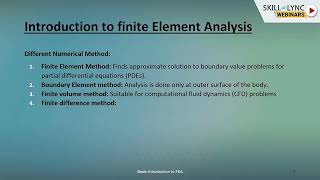Method of solving engineering problems

LOCKED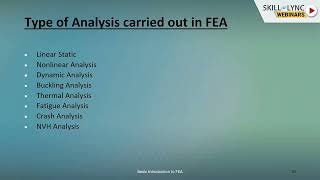Advantages and types of FEA analysis

LOCKED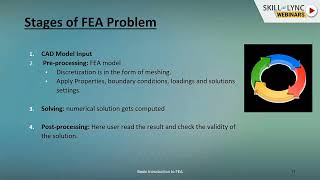Stages of FEA problem

LOCKED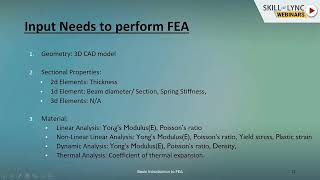Input needs to perform FEA

LOCKED

Complete the course &

Earn a CertificateLoved by our students placed atShare

Glad you have shown interest in learning Basic introduction to FEA | Skill-Lync. Share this with a friend.Close